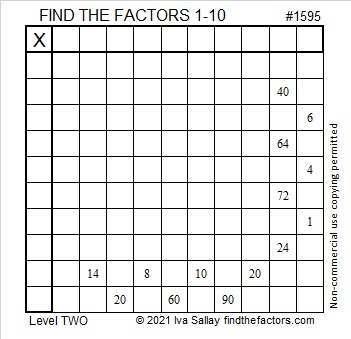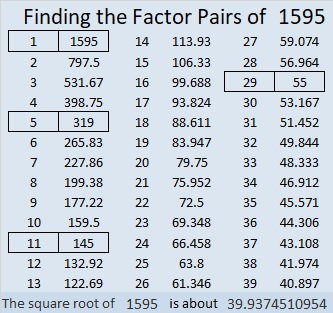# 1595 and Level 2

Contents

### Today’s Puzzle:

This puzzle is just a multiplication table whose missing factors are not in the usual order. Can you figure out where the factors from 1 to 10 should go?### Factors of 1595:

1595 ends with a 5, so it is divisible by 5.
1 – 5 + 9 – 5 = 0, so 1595 is divisible by 11.

• 1595 is a composite number.
• Prime factorization: 1595 = 5 × 11 × 29.
• 1595 has no exponents greater than 1 in its prime factorization, so √1595 cannot be simplified.
• The exponents in the prime factorization are 1, 1, and 1. Adding one to each exponent and multiplying we get (1 + 1)(1 + 1)(1 + 1) = 2 × 2 × 2 = 8. Therefore 1595 has exactly 8 factors.
• The factors of 1595 are outlined with their factor pair partners in the graphic below.### More about the Number 1595:

1595 is the hypotenuse of FOUR Pythagorean triples:
187-1584-1595, which is 11 times (17-144-145),
264-1573-1595, which is 11 times (24-143-145),
957-1276-1595, which is (3-4-5) times 319, and
1100-1155-1595, which is (20-21-29) times 55.

This site uses Akismet to reduce spam. Learn how your comment data is processed.